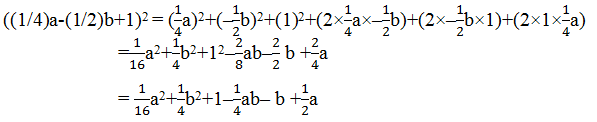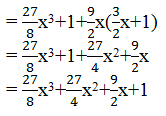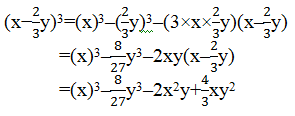# NCERT Solutions For Class 9 Math Chapter – 2 Exercise – 2.5

NCERT Solutions For Class 9 Math Chapter – 2 Exercise – 2.5

Q1. Use suitable identities to find the following products:

• (x+4)(x+10)

Solution:-

(x+4)(x+10) = x2 + (4+10)x+(4×10)

= x2 + 14x + 40

• (x+8)(x-10)

Solution:-

= x2 + (8+(-10))x +(8x(-10))

= x2 + (8-10)x – 80

= x2 – 2x – 80

• (3x+4)(3x-5)

Solution:-

Solution:

Using the identity, (x+a)(x+b) = x 2+(a+b)x+ab

[Here, x = 3x, a = 4 and b = −5]

We get,

(3x+4)(3x−5) = (3x)2+[4+(−5)]3x+4×(−5)

= 9×2+3x(4–5)–20

= 9×2–3x–20

(iv) (y2+3/2)(y2-3/2)

Solution:

Using the identity, (x+y)(x–y) = x2–y 2

[Here, x = y2and y = 3/2]

We get,

(y2+3/2)(y2–3/2) = (y2)2–(3/2)2

= y4–9/4

1. Evaluate the following products without multiplying directly:

(i) 103×107

Solution:

103×107= (100+3)×(100+7)

Using identity, [(x+a)(x+b) = x2+(a+b)x+ab

Here, x = 100

a = 3

b = 7

We get, 103×107 = (100+3)×(100+7)

=

(100)2+(3+7)100+(3×7))

= 10000+1000+21

= 11021

(ii) 95×96

Solution:

95×96 = (100-5)×(100-4)

Using identity, [(x-a)(x-b) = x2-(a+b)x+ab

Here, x = 100

a = -5

b = -4

We get, 95×96 = (100-5)×(100-4)

= (100)2+100(-5+(-4))+(-5×-4)

= 10000-900+20

= 9120

(iii) 104×96

Solution:

104×96 = (100+4)×(100–4)

Using identity, [(a+b)(a-b)= a2-b2]

Here, a = 100

b = 4

We get, 104×96 = (100+4)×(100–4)

= (100)2–(4)2

= 10000–16

= 9984

1. Factorize the following using appropriate identities:

(i) 9x2+6xy+y2

Solution:

9×2+6xy+y2 = (3x)2+(2×3x×y)+y2

Using identity, x2+2xy+y2 = (x+y)2

Here, x = 3x

y = y

9×2+6xy+y2 = (3x)2+(2×3x×y)+y2

= (3x+y)2

= (3x+y)(3x+y)

(ii) 4y2−4y+1

Solution:

4y2−4y+1 = (2y)2–(2×2y×1)+1

Using identity, x2 – 2xy + y2 = (x – y)2

Here, x = 2y

y = 1

4y2−4y+1 = (2y)2–(2×2y×1)+12

= (2y–1)2

= (2y–1)(2y–1)

(iii)  x2–y2/100

Solution:

x2–y2/100 = x2–(y/10)2

Using identity, x2-y2 = (x-y)(x+y)

Here, x = x

y = y/10

x2–y2/100 = x2–(y/10)2

= (x–y/10)(x+y/10)

1. Expand each of the following, using suitable identities:

(i) (x+2y+4z)2

(ii) (2x−y+z)2

(iii) (−2x+3y+2z)2

(iv) (3a –7b–c)2

(v) (–2x+5y–3z)2

((1/4)a-(1/2)b +1)2

Solution:

(i) (x+2y+4z)2

Using identity, (x+y+z)2 = x2+y2+z2+2xy+2yz+2zx

Here, x = x

y = 2y

z = 4z

(x+2y+4z)2 = x2+(2y)2+(4z)2+(2×x×2y)+(2×2y×4z)+(2×4z×x)

= x2+4y2+16z2+4xy+16yz+8xz

(ii) (2x−y+z)2

Using identity, (x+y+z)2 = x2+y2+z2+2xy+2yz+2zx

Here, x = 2x

y = −y

z = z

(2x−y+z)2 = (2x)2+(−y)2+z2+(2×2x×−y)+(2×−y×z)+(2×z×2x)

= 4×2+y2+z2–4xy–2yz+4xz

(iii) (−2x+3y+2z)2

Solution:

Using identity, (x+y+z)2 = x2+y2+z2+2xy+2yz+2zx

Here, x = −2x

y = 3y

z = 2z

(−2x+3y+2z)2 = (−2x)2+(3y)2+(2z)2+(2×−2x×3y)+(2×3y×2z)+(2×2z×−2x)

= 4×2+9y2+4z2–12xy+12yz–8xz

(iv) (3a –7b–c)2

Solution:

Using identity (x+y+z)2 = x2+y2+z2+2xy+2yz+2zx

Here, x = 3a

y = – 7b

z = – c

(3a –7b– c)2 = (3a)2+(– 7b)2+(– c)2+(2×3a ×– 7b)+(2×– 7b ×– c)+(2×– c ×3a)

= 9a2 + 49b2 + c2– 42ab+14bc–6ca

(v) (–2x+5y–3z)2

Solution:

Using identity, (x+y+z)2 = x2+y2+z2+2xy+2yz+2zx

Here, x = –2x

y = 5y

z = – 3z

(–2x+5y–3z)2 = (–2x)2+(5y)2+(–3z)2+(2×–2x × 5y)+(2× 5y×– 3z)+(2×–3z ×–2x)

= 4×2+25y2 +9z2– 20xy–30yz+12zx

(vi) ((1/4)a-(1/2)b+1)2

Solution:

Using identity, (x+y+z)2 = x2+y2+z2+2xy+2yz+2zx

Here, x = (1/4)a

y = (-1/2)b

z = 11. Factorize:

(i) 4x2+9y2+16z2+12xy–24yz–16xz

(ii ) 2x2+y2+8z2–2√2xy+4√2yz–8xz

Solution:

(i) 4x2+9y2+16z2+12xy–24yz–16xz

Using identity, (x+y+z)2 = x2+y2+z2+2xy+2yz+2zx

We can say that, x2+y2+z2+2xy+2yz+2zx = (x+y+z)2

4×2+9y2+16z2+12xy–24yz–16xz = (2x)2+(3y)2+(−4z)2+(2×2x×3y)+(2×3y×−4z)+(2×−4z×2x)

= (2x+3y–4z)2

= (2x+3y–4z)(2x+3y–4z)

(ii) 2x2+y2+8z2–2√2xy+4√2yz–8xz

Using identity, (x +y+z)2 = x2+y2+z2+2xy+2yz+2zx

We can say that, x2+y2+z2+2xy+2yz+2zx = (x+y+z)2

2×2+y2+8z2–2√2xy+4√2yz–8xz

= (-√2x)2+(y)2+(2√2z)2+(2×-√2x×y)+(2×y×2√2z)+(2×2√2×−√2x)

= (−√2x+y+2√2z)2

= (−√2x+y+2√2z)(−√2x+y+2√2z)

Write the following cubes in expanded form:

(i) (2x+1)3

(ii) (2a−3b)3

(iii) ((3/2)x+1)3

(iv) (x−(2/3)y)3

Solution:

(i) (2x+1)3

Using identity,(x+y)3 = x3+y3+3xy(x+y)

(2x+1)3= (2x)3+13+(3×2x×1)(2x+1)

= 8×3+1+6x(2x+1)

= 8×3+12×2+6x+1

(ii) (2a−3b)3

Using identity,(x–y)3 = x3–y3–3xy(x–y)

(2a−3b)3 = (2a)3−(3b)3–(3×2a×3b)(2a–3b)

= 8a3–27b3–18ab(2a–3b)

= 8a3–27b3–36a2b+54ab2

(iii) ((3/2)x+1)3

Using identity,(x+y)3 = x3+y3+3xy(x+y)

((3/2)x+1)3=((3/2)x)3+13+(3×(3/2)x×1)((3/2)x +1)(iv)  (x−(2/3)y)3

Using identity, (x –y)3 = x3–y3–3xy(x–y)1. Evaluate the following using suitable identities:

(i) (99)3

(ii) (102)3

(iii) (998)3

Solutions:

(i) (99)3

Solution:

We can write 99 as 100–1

Using identity, (x –y)3 = x3–y3–3xy(x–y)

(99)3 = (100–1)3

= (100)3–13–(3×100×1)(100–1)

= 1000000 –1–300(100 – 1)

= 1000000–1–30000+300

= 970299

(ii) (102)3

Solution:

We can write 102 as 100+2

Using identity,(x+y)3 = x3+y3+3xy(x+y)

(100+2)3 =(100)3+23+(3×100×2)(100+2)

= 1000000 + 8 + 600(100 + 2)

= 1000000 + 8 + 60000 + 1200

= 1061208

(iii) (998)3

Solution:

We can write 99 as 1000–2

Using identity,(x–y)3 = x3–y3–3xy(x–y)

(998)3 =(1000–2)3

=(1000)3–23–(3×1000×2)(1000–2)

= 1000000000–8–6000(1000– 2)

= 1000000000–8- 6000000+12000

= 994011992

1. Factorise each of the following:

(i) 8a3+b3+12a2b+6ab2

(ii) 8a3–b3–12a2b+6ab2

(iii) 27–125a3–135a +225a2

(iv) 64a3–27b3–144a2b+108ab2

(v) 27p3–(1/216)−(9/2) p2+(1/4)p

Solutions:

(i) 8a3+b3+12a2b+6ab2

Solution:

The expression, 8a3+b3+12a2b+6ab2 can be written as (2a)3+b3+3(2a)2b+3(2a)(b)2

8a3+b3+12a2b+6ab2 = (2a)3+b3+3(2a)2b+3(2a)(b)2

= (2a+b)3

= (2a+b)(2a+b)(2a+b)

Here, the identity, (x +y)3 = x3+y3+3xy(x+y) is used.

(ii) 8a3–b3–12a2b+6ab2

Solution:

The expression, 8a3–b3−12a2b+6ab2 can be written as (2a)3–b3–3(2a)2b+3(2a)(b)2

8a3–b3−12a2b+6ab2 = (2a)3–b3–3(2a)2b+3(2a)(b)2

= (2a–b)3

= (2a–b)(2a–b)(2a–b)

Here, the identity,(x–y)3 = x3–y3–3xy(x–y) is used.

(iii) 27–125a3–135a+225a2

Solution:

The expression, 27–125a3–135a +225a2 can be written as 33–(5a)3–3(3)2(5a)+3(3)(5a)2

27–125a3–135a+225a2 =
33–(5a)3–3(3)2(5a)+3(3)(5a)2

= (3–5a)3

= (3–5a)(3–5a)(3–5a)

Here, the identity, (x–y)3 = x3–y3-3xy(x–y) is used.

(iv) 64a3–27b3–144a2b+108ab2

Solution:

The expression, 64a3–27b3–144a2b+108ab2can be written as (4a)3–(3b)3–3(4a)2(3b)+3(4a)(3b)2

64a3–27b3–144a2b+108ab2=
(4a)3–(3b)3–3(4a)2(3b)+3(4a)(3b)2

=(4a–3b)3

=(4a–3b)(4a–3b)(4a–3b)

Here, the identity, (x – y)3 = x3 – y3 – 3xy(x – y) is used.

(v) 7p3– (1/216)−(9/2) p2+(1/4)p

Solution:

The expression, 27p3–(1/216)−(9/2) p2+(1/4)p

can be written as (3p)3–(1/6)3–3(3p)2(1/6)+3(3p)(1/6)2

27p3–(1/216)−(9/2) p2+(1/4)p =
(3p)3–(1/6)3–3(3p)2(1/6)+3(3p)(1/6)2

= (3p–16)3

= (3p–16)(3p–16)(3p–16)

1. Verify:

(i) x3+y= (x+y)(x2–xy+y2)

(ii) x3–y= (x–y)(x2+xy+y2)

Solutions:

(i) x3+y= (x+y)(x2–xy+y2)

We know that, (x+y)3 = x3+y3+3xy(x+y)

⇒ x3+y3 = (x+y)3–3xy(x+y)

⇒ x3+y3 = (x+y)[(x+y)2–3xy]

Taking (x+y) common ⇒ x3+y3 = (x+y)[(x2+y2+2xy)–3xy]

⇒ x3+y3 = (x+y)(x2+y2–xy)

(ii) x3–y= (x–y)(x2+xy+y2

We know that,(x–y)3 = x3–y3–3xy(x–y)

⇒ x3−y3 = (x–y)3+3xy(x–y)

⇒ x3−y3 = (x–y)[(x–y)2+3xy]

Taking (x+y) common ⇒ x3−y3 = (x–y)[(x2+y2–2xy)+3xy]

⇒ x3+y3 = (x–y)(x2+y2+xy)

1. Factorize each of the following:

(i) 27y3+125z3

(ii) 64m3–343n3

Solutions:

(i) 27y3+125z3

The expression, 27y3+125z3 can be written as (3y)3+(5z)3

27y3+125z3 = (3y)3+(5z)3

We know that, x3+y3 = (x+y)(x2–xy+y2)

27y3+125z3 = (3y)3+(5z)3

= (3y+5z)[(3y)2–(3y)(5z)+(5z)2]

= (3y+5z)(9y2–15yz+25z2)

(ii) 64m3–343n3

The expression, 64m3–343n3can be written as (4m)3–(7n)3

64m3–343n3 =
(4m)3–(7n)3

We know that, x3–y3 = (x–y)(x2+xy+y2)

64m3–343n3 = (4m)3–(7n)3

= (4m-7n)[(4m)2+(4m)(7n)+(7n)2]

= (4m-7n)(16m2+28mn+49n2)

1. Factorise: 27x3+y3+z3–9xyz

Solution:

The expression27x3+y3+z3–9xyz can be written as (3x)3+y3+z3–3(3x)(y)(z)

27×3+y3+z3–9xyz  = (3x)3+y3+z3–3(3x)(y)(z)

We know that, x3+y3+z3–3xyz = (x+y+z)(x2+y2+z2–xy –yz–zx)

27×3+y3+z3–9xyz  = (3x)3+y3+z3–3(3x)(y)(z)

= (3x+y+z)[(3x)2+y2+z2–3xy–yz–3xz]

= (3x+y+z)(9×2+y2+z2–3xy–yz–3xz)

1. Verify that:

x3+y3+z3–3xyz = (1/2) (x+y+z)[(x–y)2+(y–z)2+(z–x)2]

Solution:

We know that,

x3+y3+z3−3xyz = (x+y+z)(x2+y2+z2–xy–yz–xz)

⇒ x3+y3+z3–3xyz = (1/2)(x+y+z)[2(x2+y2+z2–xy–yz–xz)]

= (1/2)(x+y+z)(2×2+2y2+2z2–2xy–2yz–2xz)

= (1/2)(x+y+z)[(x2+y2−2xy)+(y2+z2–2yz)+(x2+z2–2xz)]

= (1/2)(x+y+z)[(x–y)2+(y–z)2+(z–x)2]

1. If  x+y+z = 0, show that x3+y3+z= 3xyz.

Solution:

We know that,

x3+y3+z3-3xyz = (x +y+z)(x2+y2+z2–xy–yz–xz)

Now, according to the question, let (x+y+z) = 0,

then, x3+y3+z3 -3xyz = (0)(x2+y2+z2–xy–yz–xz)

⇒ x3+y3+z3–3xyz = 0

⇒ x3+y3+z3 = 3xyz

Hence Proved

1. Without actually calculating the cubes, find the value of each of the following:

(i) (−12)3+(7)3+(5)3

(ii) (28)3+(−15)3+(−13)3

Solution:

(i) (−12)3+(7)3+(5)3

Let a = −12

b = 7

c = 5

We know that if x+y+z = 0, then x3+y3+z3=3xyz.

Here, −12+7+5=0

(−12)3+(7)3+(5)3 = 3xyz

= 3×-12×7×5

= -1260

(ii) (28)3+(−15)3+(−13)3

Solution:

(28)3+(−15)3+(−13)3

Let a = 28

b = −15

c = −13

We know that if x+y+z = 0, then x3+y3+z3 = 3xyz.

Here, x+y+z = 28–15–13 = 0

(28)3+(−15)3+(−13)3 = 3xyz

= 0+3(28)(−15)(−13)

= 16380

1. Give possible expressions for the length and breadth of each of the following rectangles, in which their areas are given:

(i) Area : 25a2–35a+12

(ii) Area : 35y2+13y–12

Solution:

(i) Area : 25a2–35a+12

Using the splitting the middle term method,

We have to find a number whose sum = -35 and product =25×12=300

We get -15 and -20 as the numbers [-15+-20=-35 and -15×-20=300]

25a2–35a+12 = 25a2–15a−20a+12

= 5a(5a–3)–4(5a–3)

= (5a–4)(5a–3)

Possible expression for length  = 5a–4

Possible expression for breadth  = 5a –3

(ii) Area : 35y2+13y–12

Using the splitting the middle term method,

We have to find a number whose sum = 13 and product = 35×-12 = 420

We get -15 and 28 as the numbers [-15+28 = 13 and -15×28=420]

35y2+13y–12 = 35y2–15y+28y–12

= 5y(7y–3)+4(7y–3)

= (5y+4)(7y–3)

Possible expression for length  = (5y+4)

Possible expression for breadth  = (7y–3)

1. What are the possible expressions for the dimensions of the cuboids whose volumes are given below?

(i) Volume : 3x2–12x

(ii) Volume : 12ky2+8ky–20k

Solution:

(i) Volume : 3×2–12x

3×2–12x can be written as 3x(x–4) by taking 3x out of both the terms.

Possible expression for length = 3

Possible expression for breadth = x

Possible expression for height = (x–4)

(ii) Volume:
12ky2+8ky–20k

12ky2+8ky–20k can be written as 4k(3y2+2y–5) by taking 4k out of both the terms.

12ky2+8ky–20k = 4k(3y2+2y–5)

[Here, 3y2+2y–5 can be written as 3y2+5y–3y–5 using splitting the middle term method.]

= 4k(3y2+5y–3y–5)

= 4k[y(3y+5)–1(3y+5)]

= 4k(3y+5)(y–1)

Possible expression for length = 4k

Possible expression for breadth = (3y +5)

Possible expression for height = (y -1)